# 使用序表与排列

## 访问

 A B 1 =file("Order_Books.txt").import@t() =A1.select(Amount>20000)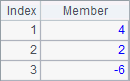B1中排列如下：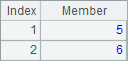A B 1 =file("Order_Books.txt").import@t() =A1.select(Amount>20000) 2 =A1(20).PName =B1(2).PName

A2B2中的结果如下：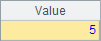field函数和array函数也可以获取序表或者记录中字段的值，如：

 A B 1 =file("Order_Books.txt").import@t() =A1.field(2) 2 =A1(20).field(2) =A1(20).array(PName) 3 =A1(20).array(PID,PName) =A1(20).array()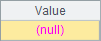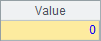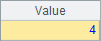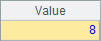A B 1 =file("Order_Books.txt").import@t() =A1.select(Amount>20000) 2 =A1.(PName) =A1.new(PName,Amount)

A2B2中的计算结果分别如下：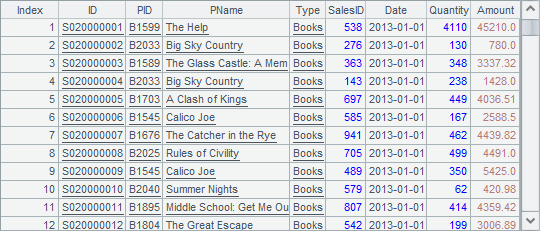T.(x)，这种语法只能取一列数据，此时的计算结果为序列，没有结构化的列名。T.new() 函数可以取一列或多列数据，此时的计算结果为序表，有结构化的列名。

 A B 1 =file("Order_Books.txt").import@t() =A1.select(Amount>20000) 2 =A1([1,2]) =B1([1,2])

A2中结果如下：B2中结果如下：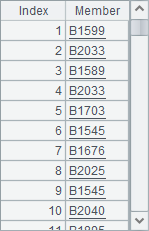## 循环函数

 A B 1 =file("Order_Books.txt").import@t() =A1.select(Amount>=20000 && month(Date)==3)

B1中计算结果如下：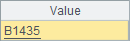A B 1 =file("Order_Books.txt").import@t() =A1.select(Amount>20000) 2 =B1.sort(SalesID,-Date)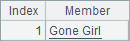## 聚合函数

Amount字段的最大值，排列和序表在这里的写法完全一样，仅以序表A1为例：

 A B 1 =file("Order_Books.txt").import@t() =A1.select(Amount>20000) 2 =A1.max(Amount)

A2中计算结果如下：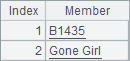A B 1 =file("Order_Books.txt").import@t() =A1.select(Amount>20000) 2 =A1.groups(SalesID, month(Date); round(sum(Amount),2), count(~))

A2中计算结果如下：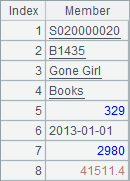## 集合间运算

 A B 1 =file("Order_Books.txt").import@t() 2 =A1.select(Amount>=20000 && month(Date)==3) =A1.select(SalesID==540 || SalesID==992) 3 =A2^B2 =A2\B2

A2中排列如下：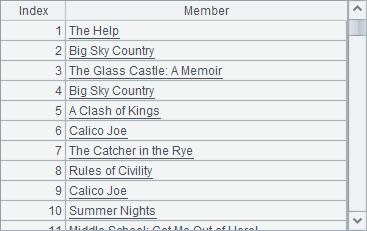B2中排列如下：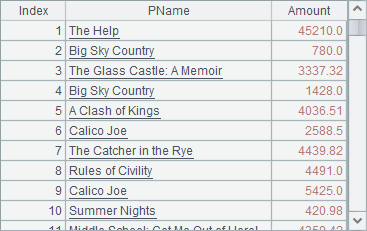A3中计算交集，结果是排列：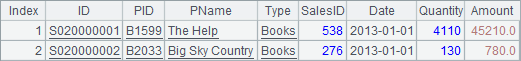B3中计算差集，从A2中去掉B2的成员，结果仍然是排列：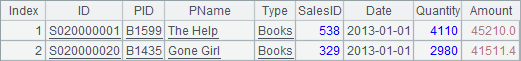A B 1 =file("Order_Books.txt").import@t() 2 =A1.select(Amount>=20000 && month(Date)==3) =A1.select(SalesID==540 || SalesID==992) 3 =A2.derive() =B2.derive() 4 =A3^B3 =A3\B3

A3B3中的序表中的数据，和前面例子中A2B2是相同的。

A4中计算A3B3的交集，结果为空集：B4中计算A3B3的差集，因为两个序表的成员总是不同的，因此差集的计算结果仍为A3A B 1 =file("Order_Books.txt").import@t() =A1.select(Amount>=20000 && month(Date)==3) 2 =B1.select(SalesID==540 || SalesID==992) =B1.select(SalesID==540 || SalesID==668) 3 =A2&B2 =A2|B2

A2中排列如下：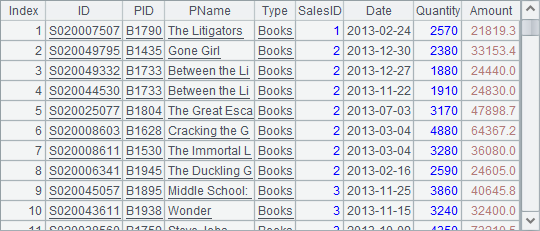B2中排列如下：A3中计算A2B2的并集。A2B2的成员会按顺序合并，重复的记录会去除。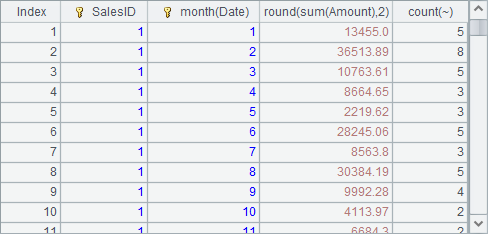A5中计算A2A3的合集。A2A3的成员会按顺序合并，重复的记录不会去除。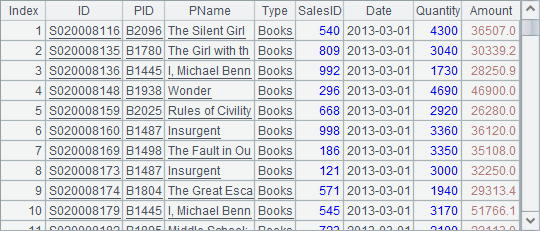A B 1 =file("Order_Books.txt").import@t() =A1.select(Amount>=20000 && month(Date)==3) 2 =B1.select(SalesID==540 || SalesID==992) =B1.select(SalesID==540 || SalesID==668) 3 =A2.derive() =B2.derive() 4 =A3&B3 =A3|B3

A3B3中的序表中的数据，和前面例子中A2B2是相同的。

A4中计算A3B3的并集。两个序表的成员完全不同，做并运算即相当于两个表的简单合并（union all），这和做合集运算的结果相同：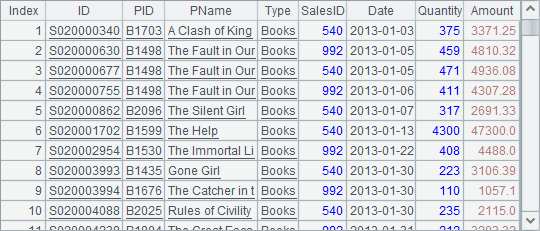B4中计算A3B3的合集，结果与A4中计算并集时完全相同：